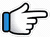# Hamari Adhuri Kahani – Keyboard Notes## Hamari Adhuri Kahani Keyboard Notes

For Hamari Adhuri Kahani Notes as Western or Indian Notations, Click –

Western –
Indian –

Lyrics –

## Keyboard Notes for Hamari Adhuri Kahani – Indian Notation (srg mpdn)

g ~        r g~
Paas     aaye

g  p n  r*~      n       p
Dooriyaan     phir   bhi
g  d s*  r*~     n       p

d~~     p       p m~~
kam    na     hui

m     g r g~~   g

p n r*~ n   p d~~ p    p m~~
hama~ri    kahaani    rahi

d n s*~      d     p d~
Aasmaan   ko   zameen,

d       n s*~  d    p d~
ye     zaroori      nahi

p d p~~ r       p m g~~
Jaa mile~      ja mile~

d n     s*~  d      p d~~
Ishq   saccha    wahi

d n        s*~  d    p d~~
Jisko     milti       nahi

p d p~~ r         p m g~~
manzilein~       manzilein~

p g* r* g*       r* g*    r* g*~
Rang thhe,      noor     thha

r*     g*m*       g* m*   g* m*~
Jab  kareeb     tu       tha

m* g*    r*~ s* r*    s* r*~
Ek        jannat       sa  tha,

p*       m* g*~~    m* g* r*~~
yeh     jahaan      ~~~~

p g* r* g*    r* g*     r* g*~
Waqt ki       ret        pe

r*         g*m*     g* m*    g* m*~
kuch    mere    naam    sa

m* g*     r*~     s* r*        s* r*~
Likh       ke     chhod     gaya

p*       m* g*~~     m* g* r*~~
tu        kahaan      ~~~

s*  n  d    d  d  n   p  p d

n d p~       p p p~    r r g~~

s*  n  d    d  d  n   p n d

n d p~     p p p~   r p m g~~